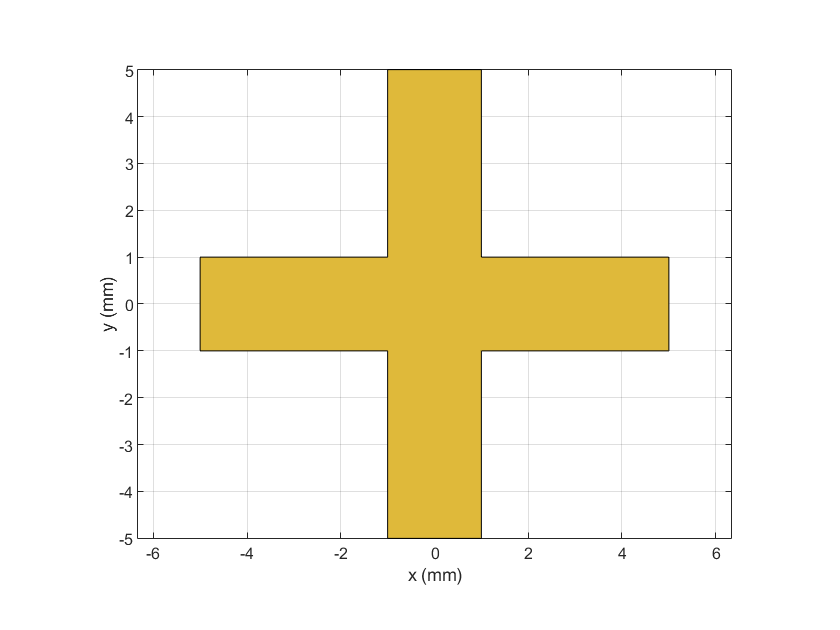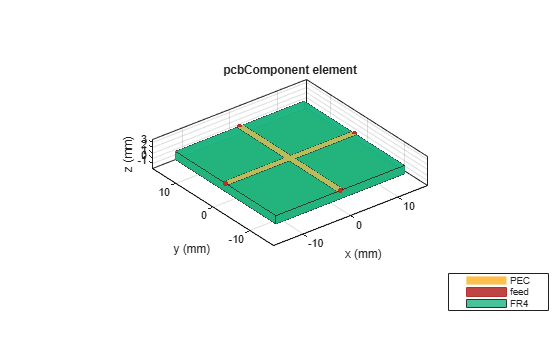# traceCross

Create cross-shaped trace

Since R2021b

## Description

Use the `traceCross` object to create a cross-shaped trace on the X-Y plane.

Note

This shape object supports behavioral modeling. For more information, see Behavioral Models.

## Creation

### Syntax

``trace = traceCross``
``trace = traceCross(Name=Value)``

### Description

example

````trace = traceCross` creates a cross-shaped trace with default properties on the X-Y plane.```
````trace = traceCross(Name=Value)` sets Properties using one or more name-value arguments. For example, `traceCross(ReferencePoint=[1 1])` creates a cross-shaped trace at the reference point `[1 1]`. Properties not specified retain their default values.```

## Properties

expand all

Name of the cross-shaped trace, specified as a character vector or a string scalar.

Example: `trace = traceCross(Name="traceCrossShape")`

Data Types: `char` | `string`

Reference point for the cross-shaped trace in Cartesian coordinates, specified as a two-element vector.

Example: `trace = traceCross(ReferencePoint=[1 1])`

Data Types: `double`

Length of the cross-shaped trace in meters, specified as a two-element vector of positive elements.

Example: `trace = traceCross(Length=[0.0800 0.0400])`

Data Types: `double`

Width of the cross-shaped trace in meters, specified as a two-element vector of positive elements.

Example: `trace = traceCross(Width=[0.005 0.005])`

Data Types: `double`

Offset along the X and Y direction in meters, specified as a two-element vector.

Example: `trace = traceCross(Offset=[1 1])`

Data Types: `double`

## Object Functions

 `add` Boolean unite operation on two RF PCB shapes `and` Shape1 & Shape2 for RF PCB shapes `area` Calculate area of RF PCB shape in square meters `intersect` Boolean intersection operation on two RF PCB shapes `mesh` Change and view mesh properties of metal or dielectric in PCB component `minus` Shape1 - Shape2 for RF PCB shapes `plus` Shape1 + Shape2 for RF PCB shapes `rotate` Rotate RF PCB shape about defined axis `rotateX` Rotate RF PCB shape about x-axis `rotateY` Rotate RF PCB shape about y-axis and angle `rotateZ` Rotate RF PCB shape about z-axis `subtract` Boolean subtraction operation on two RF PCB shapes `scale` Change size of RF PCB shape by fixed amount `show` Display PCB component structure or PCB shape `translate` Move RF PCB shape to new location

## Examples

collapse all

Create a cross-shaped trace with default properties.

`trace = traceCross`
```trace = traceCross with properties: Name: 'mytraceCross' ReferencePoint: [0 0] Length: [0.0100 0.0100] Width: [0.0020 0.0020] Offset: [0 0] ```

View the trace.

`show(trace)`Design a microstrip transmission line at 3 GHz using FR4 substrate.

```d = dielectric('FR4'); d.LossTangent = 0; m = design(microstripLine('Substrate',d),3e9,'Z0',75,... 'LineLength',0.5);```

Create a microstrip cross.

```layer2d = traceCross('Length',[m.Length m.Length], ... 'Width',[m.Width m.Width]);```

Convert the cross trace to a PCB component.

```robj = pcbComponent(layer2d); robj.BoardThickness = m.Substrate.Thickness; robj.Layers{2} = m.Substrate; show(robj)```Define frequency points to calculate the s-parameters.

`freq = (1:3:40)*100e6;`

Calculate the s-parameters of the cross trace using the behavioral model.

`Sckt = sparameters(robj,freq,'Behavioral',true);`
```Warning: Behavioral model is valid only when EpsilonR is 9.9. ```

Calculate the s-parameters of the cross trace using the electromagnetic solver.

`Sem = sparameters(robj,freq);`

References:

1. Ramesh Garg & I. J. Bahl (1978) Microstrip discontinuities, International Journal of Electronics, 45:1, 81-87, DOI: 10.1080/00207217808900883

2. Wadell, Brian C. Transmission Line Design Handbook. The Artech House Microwave Library. Boston: Artech House, 1991.

## Version History

Introduced in R2021b